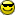## 3D Spectrum Analyser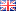More than a year ago, a friend of mine asked me to write the software for his 3D Spectrum Analyser (3DSA): a device that takes as input an audio signal, and outputs its visualisation on a 3D matrix of leds. If the above description doesn't quite ring a bell, simply watch the end result in action:

For the hardware fans amongst you, my friend documented the construction process meticulously:

It's definitely worth watching, e.g. the guy literally built his own fracking PCB from scratch!

I, however, am more a software kinda guy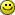In this log, I'll describe the basic building blocks we needed to get a sound spectrum analyzing algorithm going.

###### Intro
First things first though, the microprocessor to be programmed was an 80MHz Olimex PIC32, soldered to the PIC32-PINGUINO-OTG development board. (For those who ever tinkered with Arduino boards: it's the same, only with a faster chip and fewer builtin libraries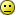) The Algorithm had to sample the input signal at regular time intervals, convert this signal to the frequency domain, and visualize the detected frequencies on a 16x16x5 LED matrix.

###### Mathematics
Of course, before writing any code, we had to figure out how to convert input samples to a frequency distribution. This thing is done all the time in signal processing by applying the Discrete Fourier Transform (DFT) to the input signal. Given a signal sampled at a constant frequency, a DFT outputs a set of amplitudes of frequency bands residing in the signal. For example, when your signal mainly consists of the middle C (or Do) tone, a DFT will assign a relatively high amplitude to the frequency band encompassing the corresponding 262 Hz frequency.

However, the human ear perceives sound logarithmically, meaning that a doubling of the frequency of a sound signal is perceived only as a linearly higher tone. In order to compensate for this, we used the Constant Q transform (CQT) instead of the DFT. In short, where a DFT returns amplitudes for frequency bands f-2f-3f-4f-etc., a CQT works with frequency bands f-2f-4f-8f-etc.

So from a theoretical perspective, the algorithm needed for the 3DSA was quite simple: sample the input signal at regular time intervals, apply a CQT calculating amplitudes for 16 frequency bands, and make each of the 16 led columns blink appropriately. Given that the Pinguino development board supported C, we assumed implementing this algorithm wouldn't be that hard. However, some challenges always pop up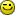###### Interrupts: a poor man's multithreading
First obstacle: how do you sample a signal at regular time intervals if you only have one thread? A simple solution would be to take a sample, calculate the CQT and visualization, and let the thread sleep until a certain time period has passed before starting a new sample-calculte-visualize cycle. However, we wanted our sampling rate to be 14 KHz, which on an 80 MHz microprocessor left les than 6k clock cycles between samples to calculate the CQT. This proved insufficient -- in the end we used ~1M clock cycles for each calculate-visualize cycle, so we had to figure out how to take new samples while doing the CQT calculation and LED visualization for old samples.

After perusing many a Pinguino forum, the solution arrived in the form of interrupts: a piece of code that has a higher priority to run than other code, and is executed by the processor at designated time intervals. Since the Pinguino devs did not provide a C library for interrupts on the PIC32, we had to manually implement this by setting some processor bits to the right value. Having grown up a Java programmer, I could almost feel the silicon under our code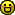###### Floating points are slow (without hardware support)
The other large obstacle turned out to be the non-existing floating point capabilities of the PIC32 chip. Doing any floating point arithmetic in the inner loop of our CQT implementation slowed down the code by an order of magnitude, turning the LED visualization in a slideshow (now I know how an old GPU must feel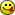). In order to remedy this, we resorted to an improvised fixed-point number format, using 10 fractional bits. It complicated multiplication a bit, but got the job done.

On a related note, at the heart of a CQT lie a sine and cosine calculation, for which the builtin functions also were way too slow. The fix was to implement our own third order Taylor expansion approximation for the sine function, based on an excellent blog of Jasper Vijn. Coincidentally, he also used fixed-point arithmetic, so that was a natural fit.
Seeing our hand-written bit optimizations speed up our algorithm by a factor 10 was ofcourse quite satisfying###### Conclusion
In the end, our code could sample the input signal at 14 kHz, calculate frequency amplitudes in the range of 20 - 7000 Hz, and get a refresh rate of 80 FPS on the PIC32 chip, meaning each CQT-visualization loop took only about 12 ms. It definitely was an instructive hobby project, would program again